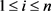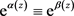﻿ 与差分方程相关的唯一性问题

# 与差分方程相关的唯一性问题Uniqueness Problem of Merpmorphic Function Concerning Difference Equation

Abstract: This paper studied the uniqueness of a finite order meromorphic solution f(z) of the difference equation f(z+c)-f(z)=R(z) sharing 0, 1, ∞ CM with meromorphic g(z). Our results sup-plemented and improved the result due to Cui and Chen in 2017.

1. 引言及主要结果

$f\left(z\right)$$g\left(z\right)$ 是定义在整个复平面上的亚纯函数，a是一个有穷复数。如果 $f\left(z\right)-a$$g\left(z\right)-a$ 有相同的零点(计重数)，则称 $f\left(z\right)$$g\left(z\right)$ CM分担a。如果 $f\left(z\right)$$g\left(z\right)$ 有相同的极点(计重数)，则称 $f\left(z\right)$$g\left(z\right)$ CM分担 $\infty$。(本中的亚纯函数均指定义在整个复平面上的亚纯函数)。

1929年，Nevanlinna  证明了著名的五值唯一性定理。

2017年，崔宁、陈宗煊  研究了一类差分方程解f与一个亚纯函数g CM分担三个值的唯一性问题，他们证明了如下定理。

${a}_{1}\left(z\right)f\left(z+1\right)+{a}_{0}\left(z\right)f\left(z\right)=F\left(z\right)$ (1.1)

(I) $f\left(z\right)\equiv g\left(z\right)$

(II) $f\left(z\right)+g\left(z\right)\equiv f\left(z\right)g\left(z\right)$

(III) 存在一个多项式 $\beta \left(z\right)=az+{b}_{0}$ 和一个常数 ${a}_{0}$ 满足 ${\text{e}}^{{a}_{0}}\ne {\text{e}}^{{b}_{0}}$，使得 $f\left(z\right)=\frac{1-{\text{e}}^{\beta \left(z\right)}}{{\text{e}}^{\beta \left(z\right)}\left({\text{e}}^{{a}_{0}-{b}_{0}}-1\right)}$$f\left(z\right)=\frac{1-{\text{e}}^{\beta \left(z\right)}}{1-{\text{e}}^{{b}_{0}-{a}_{0}}}$，其中 $a\ne 0$${b}_{0}$ 为常数。

$f\left(z+c\right)-f\left(z\right)=R\left(z\right)$ (1.2)

(1) $f\left(z\right)\equiv g\left(z\right)$

(2) $f\left(z\right)={\text{e}}^{bz+{b}_{0}}+1$$g\left(z\right)={\text{e}}^{-bz-{b}_{0}}+1$。其中 ${\text{e}}^{b}\ne 1$${a}_{1}\left(z\right)+{a}_{0}\left(z\right)\overline{)\equiv }0$

(3) 存在一个多项式 $\beta \left(z\right)=az+{b}_{0}$ 和一个常数 ${a}_{0}$ 满足 ${\text{e}}^{{a}_{0}}\ne {\text{e}}^{{b}_{0}}$，使得 $f\left(z\right)=\frac{1-{\text{e}}^{\beta \left(z\right)}}{{\text{e}}^{\beta \left(z\right)}\left({\text{e}}^{{a}_{0}-{b}_{0}}-1\right)}$$g\left(z\right)=\frac{1-{\text{e}}^{\beta \left(z\right)}}{1-{\text{e}}^{{b}_{0}-{a}_{0}}}$，其中 $a\ne 0$${b}_{0}$ 为常数。

2. 一些引理

$T\left(r,f\left(z+c\right)\right)=T\left(r,f\right)+S\left(r,f\right)$

(1) $\underset{i=1}{\overset{n}{\sum }}{f}_{i}\left(z\right){\text{e}}^{{g}_{i}\left(z\right)}\equiv 0$

(2) 对任意的$1\le k 时，均有

$T\left(r,{f}_{j}\right)=S\left(r,{\text{e}}^{{g}_{h}-{g}_{l}}\right)$$r\to \infty$$r\notin E$

${f}_{i}\left(z\right)\equiv 0\left(1\le i\le n\right)$

3. 定理3的证明

$f\left(z\right)$ CM分担0，1， $\infty$，结合引理2.3可得，存在多项式 $\alpha \left(z\right)$$\beta \left(z\right)$，使得下式成立：

$\frac{g\left(z\right)}{f\left(z\right)}={\text{e}}^{\alpha \left(z\right)}$$\frac{g\left(z\right)-1}{f\left(z\right)-1}={\text{e}}^{\beta \left(z\right)}$(3.1)，从(3.1)式，易得 $f\left(z\right)\equiv g\left(z\right)$。以下讨论 ${\text{e}}^{\alpha \left(z\right)}\overline{)\equiv }{\text{e}}^{\beta \left(z\right)}$ 的情形。

$f\left(z\right)=\frac{1-{\text{e}}^{\beta \left(z\right)}}{{\text{e}}^{\alpha \left(z\right)}-{\text{e}}^{\beta \left(z\right)}}$(3.2)

${A}_{1}{\text{e}}^{\alpha +\beta }+{A}_{2}{\text{e}}^{2\beta }+{A}_{3}{\text{e}}^{2\alpha }+{A}_{4}{\text{e}}^{\alpha }+{A}_{5}{\text{e}}^{\beta }\equiv 0$(3.3)

${B}_{2}{\text{e}}^{2\alpha }+{B}_{1}{\text{e}}^{\alpha }+{B}_{0}\equiv 0$(3.4)

$\left\{\begin{array}{l}{B}_{2}=-R\cdot {\text{e}}^{{\Delta }_{c}\alpha };\\ {B}_{1}=\left[\left(R-1\right){\text{e}}^{{\Delta }_{c}\beta }+\left(R+1\right){\text{e}}^{{\Delta }_{c}\alpha }\right]{\text{e}}^{\beta }+\left(1-{\text{e}}^{{\Delta }_{c}\alpha }\right);\\ {B}_{0}=\left({\text{e}}^{{\Delta }_{c}\beta }-1\right){\text{e}}^{\beta }-R\cdot {\text{e}}^{{\Delta }_{c}\beta }{\text{e}}^{2\beta }.\end{array}$

${C}_{2}{\text{e}}^{2\beta }+{C}_{1}{\text{e}}^{\beta }+{C}_{0}\equiv 0$(3.5)

$\left\{\begin{array}{l}{C}_{2}=-R\cdot {\text{e}}^{{\Delta }_{c}\beta };\\ {C}_{1}=\left[\left(R-1\right){\text{e}}^{{\Delta }_{c}\beta }+\left(R+1\right){\text{e}}^{{\Delta }_{c}\alpha }\right]{\text{e}}^{\alpha }+\left({\text{e}}^{{\Delta }_{c}\beta }-1\right);\\ {C}_{0}=\left(1-{\text{e}}^{{\Delta }_{c}\alpha }\right){\text{e}}^{\alpha }-R\cdot {\text{e}}^{{\Delta }_{c}\alpha }{\text{e}}^{2\alpha }.\end{array}$

${A}_{1}{\text{e}}^{{F}_{1}}+{A}_{2}{\text{e}}^{{F}_{2}}+{A}_{3}{\text{e}}^{{F}_{3}}+{A}_{4}{\text{e}}^{{F}_{4}}+{A}_{5}{\text{e}}^{{F}_{5}}\equiv 0$(3.6)

${D}_{2}{\text{e}}^{2\beta }+{D}_{1}{\text{e}}^{\beta }\equiv 0$(3.7)

$\mathrm{deg}\alpha =\mathrm{deg}\beta \ge 1$，显然 $\mathrm{deg}{\Delta }_{c}\alpha =\mathrm{deg}{\Delta }_{c}\beta =\mathrm{deg}\alpha -1$，可得 $\mathrm{deg}{p}_{1}\le \mathrm{deg}{\Delta }_{c}\alpha =\mathrm{deg}{\Delta }_{c}\beta$

${D}_{2}=-\left(R-1\right){\text{e}}^{2{\Delta }_{c}\beta }-\left(R+1\right)-2R\cdot {\text{e}}^{{\Delta }_{c}\beta }\equiv 0$(3.8)

$\text{deg}{\Delta }_{c}\beta =\text{deg}{\Delta }_{c}\alpha \ge 1$，R是有理函数，以及引理2.2，可得 $R\equiv 0$，这与R是非零有理函数相矛盾。

$f\left(z\right)=\frac{1-{\text{e}}^{{a}_{1}z+{b}_{0}}}{{\text{e}}^{{a}_{1}z+{a}_{0}}-{\text{e}}^{{a}_{1}z+{b}_{0}}}=\frac{1-{\text{e}}^{\beta \left(z\right)}}{{\text{e}}^{\beta \left(z\right)}\left({\text{e}}^{{a}_{0}-{b}_{0}}-1\right)}$

$R\left(z\right)\left({\text{e}}^{{a}_{0}-{b}_{0}}-1\right)+\left({\text{e}}^{{a}_{1}c}-1\right){\text{e}}^{\beta \left(z\right)}\equiv 0$

${G}_{4}{\text{e}}^{4\alpha }+{G}_{3}{\text{e}}^{3\alpha }+{G}_{2}{\text{e}}^{2\alpha }+{G}_{1}{\text{e}}^{\alpha }\equiv 0$(3.9)

${H}_{4}{\text{e}}^{4\beta }+{H}_{3}{\text{e}}^{3\beta }+{H}_{2}{\text{e}}^{2\beta }+{H}_{1}{\text{e}}^{\beta }\equiv 0$(3.10)

4. 定理4的证明

$f\left(z\right)=\frac{1-{\text{e}}^{2\alpha \left(z\right)}}{{\text{e}}^{\alpha \left(z\right)}-{\text{e}}^{2\alpha \left(z\right)}}={\text{e}}^{-\alpha \left(z\right)}+1$(4.1)

$\left({a}_{1}\left(z\right){\text{e}}^{-{\Delta }_{1}\alpha \left(z\right)}+{a}_{0}\left(z\right)\right){\text{e}}^{-\alpha \left(z\right)}+{a}_{1}\left(z\right)+{a}_{0}\left(z\right)-F\left(z\right)=0$(4.2)

 杨乐. 值分布及其新研究[M]. 北京: 科学出版社, 1982: 5-13.

 Yang, C.C. and Yi, H.X. (2003) Uniqueness Theory of Meromorphic Functions. Science Press, Beijing, 1-13.

 Hayman, W.K. (1964) Mermorphic Functions. Clarendon Press, Oxford, 4-10.

 Nevanlinna, R. (1929) Théorème de Picard-Borel et la théorie des fonctions méromorphes. Gauthier-Villars, Paris.
https://doi.org/10.1007/BF02592680

 Li, Y.H. and Qiao, J.Y. (2000) The Uniqueness of Meromorphic Functions Concerning Small Functions. Science in China, Series A, 43, 581-590.
https://doi.org/10.1007/BF02908769

 Li, P. and Yang, C.C. (2001) Uniqueness Theorems on Entire Functions and Their Derivatives. Journal of Mathematical Analysis and Applications, 253, 50-57.
https://doi.org/10.1006/jmaa.2000.7007

 Chen, Z.X. and Yi, H.X. (2013) On Sharing Values of Meromorphic Functions and Their Differences. Results in Mathematics, 63, 557-565.

 Heittokangas, J., Korhonen, R., Laine, I., Rieppo, J. and Zhang, J.L. (2009) Value Sharing Results for Shifts of Meromorphic Functions, and Sufficient Conditions for Periodicity. Journal of Mathematical Analysis and Applications, 355, 352-363.
https://doi.org/10.1016/j.jmaa.2009.01.053

 崔宁, 陈宗煊. 一类线性差分方程的亚纯解与一个亚纯函数分担3个值的唯一性[J]. 数学年刊, 2017，38A(1): 13-22.

 Chiang, Y.M. and Feng, S.J. (2008) On the Nevanlinna Characteristic of f(z+η) and Difference Equations in the Complex Plane. The Ramanujan Journal, 16, 105-129.
https://doi.org/10.1007/s11139-007-9101-1

Top